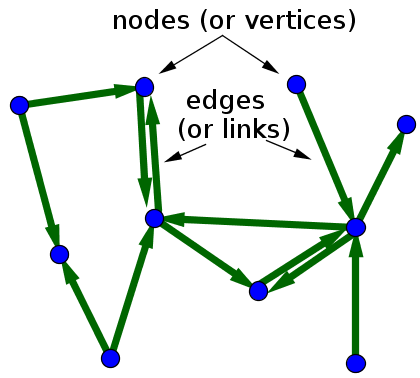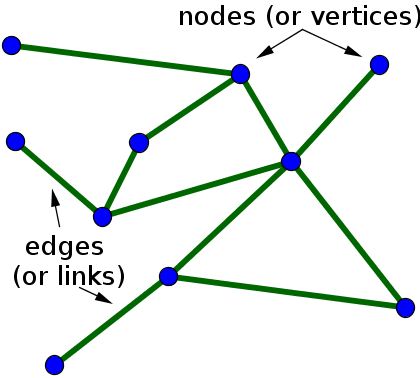# Math Insight

### Network definition

A network is a set of objects (called nodes or vertices) that are connected together. The connections between the nodes are called edges or links. In mathematics, networks are often referred to as graphs (which must be distinguished from an alternative use of the graph to mean a graph of a function).

If the edges in a network are directed, i.e., pointing in only one direction, the network is called a directed network (or a directed graph, sometimes digraph for short). When drawing a directed network, the edges are typically drawn as arrows indicating the direction, as illustrated in the first figure, below. If all edges are bidirectional, or undirected, the network is an undirected network (or undirected graph), as illustrated by the second figure.A directed network with 10 nodes (or vertices) and 13 edges (or links).An undirected network with 10 nodes (or vertices) and 11 edges (or links).

One can formally define an undirected graph as $G= (\mathcal{N},\mathcal{E})$, consisting of the set $\mathcal{N}$ of nodes and the set $\mathcal{E}$ of edges, which are unordered pairs of elements of $\mathcal{N}$. The formal definition of a directed graph is similar, the only difference is that the set $\mathcal{E}$ contains ordered pairs of elements of $\mathcal{N}$.